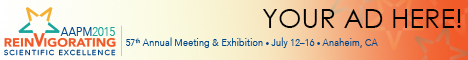# Program Information

## Reconstruction of CT Images From Sparsely-Sampled Data Using the Logarithmic Barrier MethodH Xu

## Presentations

SU-E-I-45 Sunday 3:00PM - 6:00PM Room: Exhibit Hall

Purpose: To develop and investigate whether the logarithmic barrier (LB) method can result in high-quality reconstructed CT images using sparsely-sampled noisy projection data

Methods: The objective function is typically formulated as the sum of the total variation (TV) and a data fidelity (DF) term with a parameter Î» that governs the relative weight between them. Finding the optimized value of Î» is a critical step for this approach to give satisfactory results. The proposed LB method avoid using Î» by constructing the objective function as the sum of the TV and a log function whose augment is the DF term. Newtonâ€™s method was used to solve the optimization problem. The algorithm was coded in MatLab2013b. Both Shepp-Logan phantom and a patient lung CT image were used for demonstration of the algorithm. Measured data were simulated by calculating the projection data using radon transform. A Poisson noise model was used to account for the simulated detector noise. The iteration stopped when the difference of the current TV and the previous one was less than 1%.

Results: Shepp-Logan phantom reconstruction study shows that filtered back-projection (FBP) gives high streak artifacts for 30 and 40 projections. Although visually the streak artifacts are less pronounced for 64 and 90 projections in FBP, the 1D pixel profiles indicate that FBP gives noisier reconstructed pixel values than LB does. A lung image reconstruction is presented. It shows that use of 64 projections gives satisfactory reconstructed image quality with regard to noise suppression and sharp edge preservation.

Conclusion: This study demonstrates that the logarithmic barrier method can be used to reconstruct CT images from sparsely-amped data. The number of projections around 64 gives a balance between the over-smoothing of the sharp demarcation and noise suppression. Future study may extend to CBCT reconstruction and improvement on computation speed.

Contact Email: